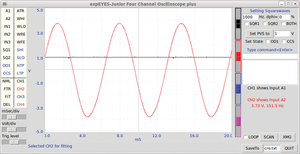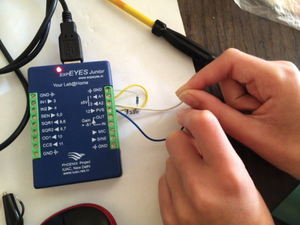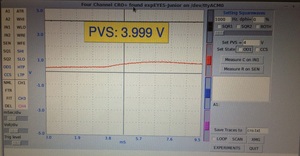# Trying out the ExpEYES science and experimentation kit

#### A Selection of Interesting Experiments

I will describe a few basic experiments to display the sort of things the ExpEYES kit can do, and you are free to dream up more.

One of the most basic things you can do is observe AC (alternating current) and DC (direct current) voltages in the oscilloscope. To do this, connect a wire between PVS and A1 on the ExpEYES board and another wire between SINE and A2, as shown in Figure 7.Figure 7: Configuring the ExpEYES Junior main board for the voltage experiment.

On the ExpEYES control panel, "drag" A1 to CH1 (black) and A2 to CH2 (red). Set PVS to 1V, and you should see output similar to Figure 8.Figure 8: Measuring voltage with the oscilloscope.

As seen on the bottom right-hand side of this window, the voltage from CH1 is shown as 1V and the sine wave on CH2 is shown as 3.73V at 151.5Hz. As a comparison, a mains power supply will have a voltage of somewhere around 100 to 250V and a frequency of 50 to 60Hz. On this screen, you can adjust the horizontal scale of the plot by dragging the mSec/div slider left or right, and you can adjust the vertical scale by dragging the Volt/div slider to the left or right.

#### Resistance of the Human Body

An interesting experiment to undertake with this kit it to measure how the resistance of your skin and body varies over time. This is very easy to set up on the ExpEYES kit using just three pieces of wire and a 200kOhm resistor. You need to connect a wire between A1 and PVS, connect the resistor between A2 and ground, and then connect an open-ended wire out of both PVS and A2. The finished setup should look Figure  9.Figure 9: Measuring resistance of the human body.

On the ExpEYES control panel, you need to drag A1 to CH1 and A2 to CH2 and set PVS to 4V. You can then pick up one of the open-ended wires in each hand and watch the response on the ExpEYES control panel. You should see something like Figure  10.Figure 10: A simple measurement is all you need to calculate resistance.

You can observe from this plot that the input on CH1 is coming out at 3.999V (which is great because it was set to 4V!). It is easy now to work out the resistance of your body using some simple equations to compare the two resistors (the 200kOhm and your body). The voltage on A2 (CH2) is showing as approximately 0.9V, and this implies that the voltage across the unknown resistor value (your body) is 4  –  0.9  = 3.1V. Using Ohm's law, you can then calculate the current, I, across the known resistor value:

I = voltage/resistance  = 0.9/200,000  = 0.0000045A  = 4.5µA

Using this value, you can then calculate the resistance of the body using the same Ohm's law equation in reverse:

R  = voltage/current = 3.1/0.0000045  = 688,889Ohm = 689kOhm

This is a surprisingly high value; however, the resistance of the human body depends hugely on how dry your skin is and a large number of other factors (body resistance is usually in the range of 1,000 to 100,000Ohm). I have a feeling this is an incorrect result, potentially from a loose connection somewhere; however, the theory still stands correct.

ExpEYES is capable of more than has been shown here – Fourier transforms, capacitance measurements, and a whole host of other measurements and experiments from the simple to the quite complicated (in theoretical terms); however, the ExpEYES kit makes them an absolute pleasure to attempt. Take a look at the experiments listed on the main page of the ExpEYES website  just below the introduction for some inspiration on where to go next.

Express-Checkout as PDF

Pages: 6

Price \$2.95
(incl. VAT)